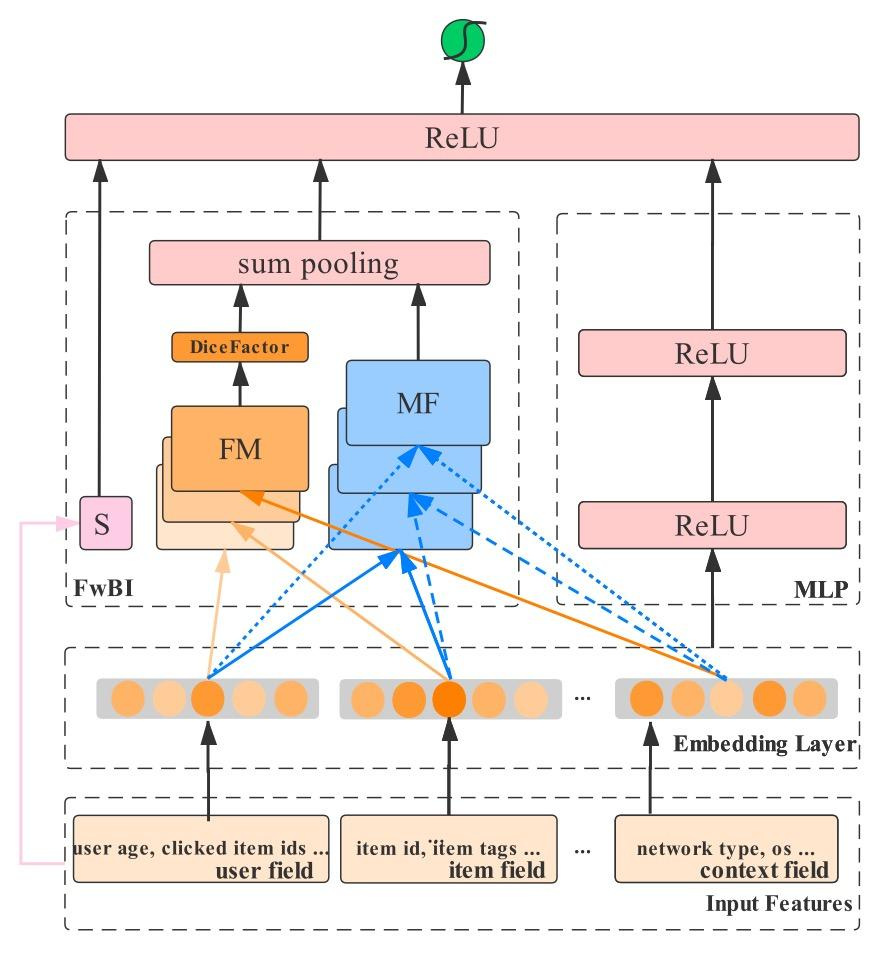## 背景

FLEN使用了一个filed-wise bi-interaction pooling技术。通过利用特征所属的场的信息，filed-wise bi-interaction pooling能够以更少的模型参数量和更短的计算时间消耗来同时捕获inter-filed和intra-filed之间的特征交互。

FLEN也是首次公开提出的能够将不同的浅层模型如FM,MF,FwFM通过一个统一的框架表达的模型。

## 关于梯度耦合问题

• 假设有如下表达式其中为FM模型的隐向量
• 利用反向传播算法更新隐向量时，关于的梯度为• 如何解决这个问题

FFM模型提出了利用特征所属的场的信息来缓解梯度耦合方法，每个特征与不同场下的特征交互时都使用了不同的隐向量。FFM及基于FFM的神经网络方法虽然能够缓解梯度耦合问题，但是其带来的参数量的增加和训练时间的延长使得其难以被大规模部署，下面就看看本文提出的FLEN是如何以一种时空高效的方法缓解该问题的。

## Embedding Layer## Field-Wise Bi-Interaction Component

• 所有特征线性组合以及全局偏置项
• 特征大类之间的交叉组合MF
• 特征大类内部的交叉组合FM

## FM模块表达的是每个大类特征组自身的相对重要性

### Field-Wise Bi-Interaction的输出

MF和FM组件的输出分别是包含了特征组之间和特征组内部特征交叉的维的向量。FwBI组件之所以能缓解梯度耦合问题，是因为其将传统的所有特征直接进行交互组合的方式变为了分组交互的方式。

## Prediction Layer## Dicefactor:Dropout Technique## DeepCTR样例

https://github.com/shenweichen/DeepCTR/github.com
import pandas as pd
from sklearn.metrics import log_loss, roc_auc_score
from sklearn.model_selection import train_test_split
from sklearn.preprocessing import LabelEncoder

from deepctr.inputs import SparseFeat, get_feature_names
from deepctr.models import FLEN

if __name__ == "__main__":
data['day'] = data['hour'].apply(lambda x: str(x)[4:6])
data['hour'] = data['hour'].apply(lambda x: str(x)[6:])

sparse_features = ['hour', 'C1', 'banner_pos', 'site_id', 'site_domain',
'site_category', 'app_id', 'app_domain', 'app_category', 'device_id',
'device_model', 'device_type', 'device_conn_type',  # 'device_ip',
'C14',
'C15', 'C16', 'C17', 'C18', 'C19', 'C20', 'C21', ]

data[sparse_features] = data[sparse_features].fillna('-1', )
target = ['click']

# 1.Label Encoding for sparse features,and do simple Transformation for dense features
for feat in sparse_features:
lbe = LabelEncoder()
data[feat] = lbe.fit_transform(data[feat])

# 2.count #unique features for each sparse field,and record dense feature field name
# 注明每个特征所属的field信息
field_info = dict(C14='user', C15='user', C16='user', C17='user',
C18='user', C19='user', C20='user', C21='user', C1='user',
banner_pos='context', site_id='context',
site_domain='context', site_category='context',
app_id='item', app_domain='item', app_category='item',
device_model='user', device_type='user',
device_conn_type='context', hour='context',
device_id='user'
)
# 在构造特征列的时候指定传入上面的field信息
fixlen_feature_columns = [
SparseFeat(name, vocabulary_size=data[name].nunique(), embedding_dim=16, use_hash=False, dtype='int32',
group_name=field_info[name]) for name in sparse_features]

dnn_feature_columns = fixlen_feature_columns
linear_feature_columns = fixlen_feature_columns

feature_names = get_feature_names(linear_feature_columns + dnn_feature_columns)

# 3.generate input data for model

train, test = train_test_split(data, test_size=0.2)
train_model_input = {name: train[name] for name in feature_names}
test_model_input = {name: test[name] for name in feature_names}

# 4.Define Model,train,predict and evaluate
# 调用FLEN模型即可
metrics=['binary_crossentropy'], )

history = model.fit(train_model_input, train[target].values,
batch_size=256, epochs=10, verbose=2, validation_split=0.2, )
pred_ans = model.predict(test_model_input, batch_size=256)
print("test LogLoss", round(log_loss(test[target].values, pred_ans), 4))
print("test AUC", round(roc_auc_score(test[target].values, pred_ans), 4))


## 参考资料

https://github.com/aimetrics/jarvisgithub.com